# Keras Data Augmentation for Scikit-learn

Here is a method to integrate a preprocessing utility from Keras with a model from Scikit-learn. The complete Jupyter notebook is in the reference section below.

We will be working with the MNIST data set:

• 60,000 Examples for the training set.
• 10,000 Examples for the test set.
• 784 Features (Images of handwritten digits, 28 * 28 pixels).

We will use a KNeighborsClassifier, a lazy learner! It memories the data during training time and learns during runtime.

Here we will use the ImageDataGenerator class from Keras. First, we create an object:

`datagen = ImageDataGenerator(    width_shift_range= 2.0,    height_shift_range= 2.0,    rotation_range = 20,    fill_mode = 'constant',)`

This object will:

• Shift the image by 2 pixels vertically and horizontally.
• Rotates the image by 20 degrees.

Calling the fit method:

`datagen.fit(X_train.reshape(X_train.shape, 28, 28, 1))`

The fit method expects a rank 4 sample as an input (Examples, width, height, channels {1 for grayscale, 3 for RGB…}).

Then we call the flow method to generate the new data set:

`data_generator = datagen.flow(X_train.reshape(X_train.shape, 28, 28, 1),shuffle=False, batch_size=1)`

We need to preserve the order of the data so that it matches the labels. The batch size should be 1 to get the whole data set.

`type(data_generator)  # => NumpyArrayIterator`

The new data is an iterator, ideal for incremental learners, but our model expects an array-like list.

We have to materialize the iterator to using the comprehension list:

`X_train_aug = [data_generator.next() for i in range(0, m * 4)]`

This step will turn the iterator into a list. The 4 is a multiplier for how many sets to generate (4 * 60k = 240k).

The last step is to reshape the data from rank 4 to its original shape of rank 2:

`X_train_240k = np.asarray(X_train_aug).reshape(m * 4, 28 * 28)`

When we plot, we get this.

Now the data is ready for a second run!

--

--

--

## More from Mansoor Aldosari

https://github.com/booletic

Love podcasts or audiobooks? Learn on the go with our new app.

## Choosing the Right Model for Object Detection## Classification- Logistic Regression## A Complete Guide To Sentiment Analysis And Its Applications## Overview of GANs (Generative Adversarial Networks) - Part I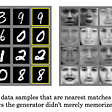## Are you evaluating your model performance correctly?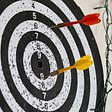## Map-Reduce and Data Parallelism## Zero to ML Hero in 5 days: Credit Card Fraud Detection — Day 2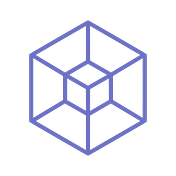## Mansoor Aldosari

https://github.com/booletic

## Types of Cross Validations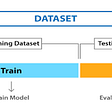## K-Nearest Neighbor in Machine Learning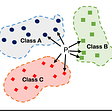## Heatmap For Correlation Matrix & Confusion Matrix | Extra Tips On Machine Learning